jtse

•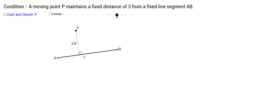Equidistance between line segment

Activity

jtse

•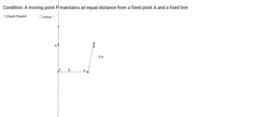Equidistance between one point and one straight line

Activity

jtse

•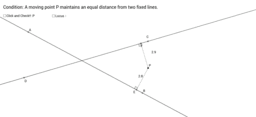Equidistance between two non-parallel Line

Activity

jtse

•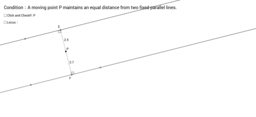Equidistance between Two Parallel line

Activity

jtse

•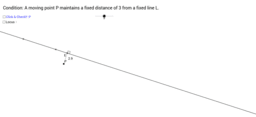Equidistance between a straight line

Activity

jtse

•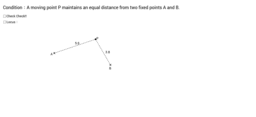Equidistance Between two point

Activity

jtse

•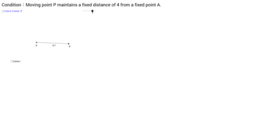Activity

jtse